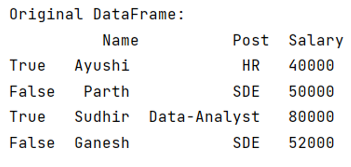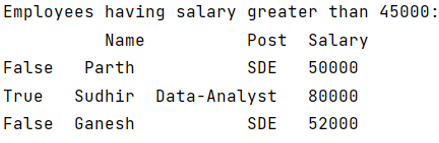# How to apply logical operators for Boolean indexing in Pandas?

Given a Pandas DataFrame, we have to How to apply logical operators for Boolean indexing.
Submitted by Pranit Sharma, on May 27, 2022

Indexing in Pandas:

Index in pandas is just the number of rows defined in a Series or DataFrame. The index always starts from 0 to n-1 where n is the number of rows. The index is used in indexing which is a method of traversing or selecting particular rows and columns or selecting all the rows and columns.

Boolean Indexing

In programming, we sometimes use some specific values that only have two values, either True or False. These values are known as Boolean values. How to apply logical operators for Boolean indexing in Pandas? is nothing but indexing the rows of the pandas DataFrame with their actual values (True or False) rather than naming them with a string or an integer value.

To achieve Boolean indexing, we simply assign a list of Boolean values to the index values while defining a DataFrame.

To work with pandas, we need to import pandas package first, below is the syntax:

```import pandas as pd
```

Let us understand with the help of an example.

```# Importing pandas package
import pandas as pd

# Creating a dictionary
d = {
'Name':["Ayushi", "Parth", "Sudhir", "Ganesh"],
'Post': ["HR", "SDE", "Data-Analyst", "SDE"],
'Salary':[40000, 50000, 80000, 52000]
}

# Creating a DataFrame
df = pd.DataFrame(d, index = [True, False, True, False])

# Display original DataFrame
print("Original DataFrame:\n",df,"\n")
```

Output:Now we will apply a logical operator in this pandas DataFrame having Boolean indexing.

```# Filter all the subsets where salary is
# greater than 45000
result = df.loc[df['Salary']>45000]

# Display result
print("Employees having salary greater than 45000:\n",result)
```

Output:Languages: » C » C++ » C++ STL » Java » Data Structure » C#.Net » Android » Kotlin » SQL
Web Technologies: » PHP » Python » JavaScript » CSS » Ajax » Node.js » Web programming/HTML
Solved programs: » C » C++ » DS » Java » C#
Aptitude que. & ans.: » C » C++ » Java » DBMS
Interview que. & ans.: » C » Embedded C » Java » SEO » HR
CS Subjects: » CS Basics » O.S. » Networks » DBMS » Embedded Systems » Cloud Computing
» Machine learning » CS Organizations » Linux » DOS
More: » Articles » Puzzles » News/Updates